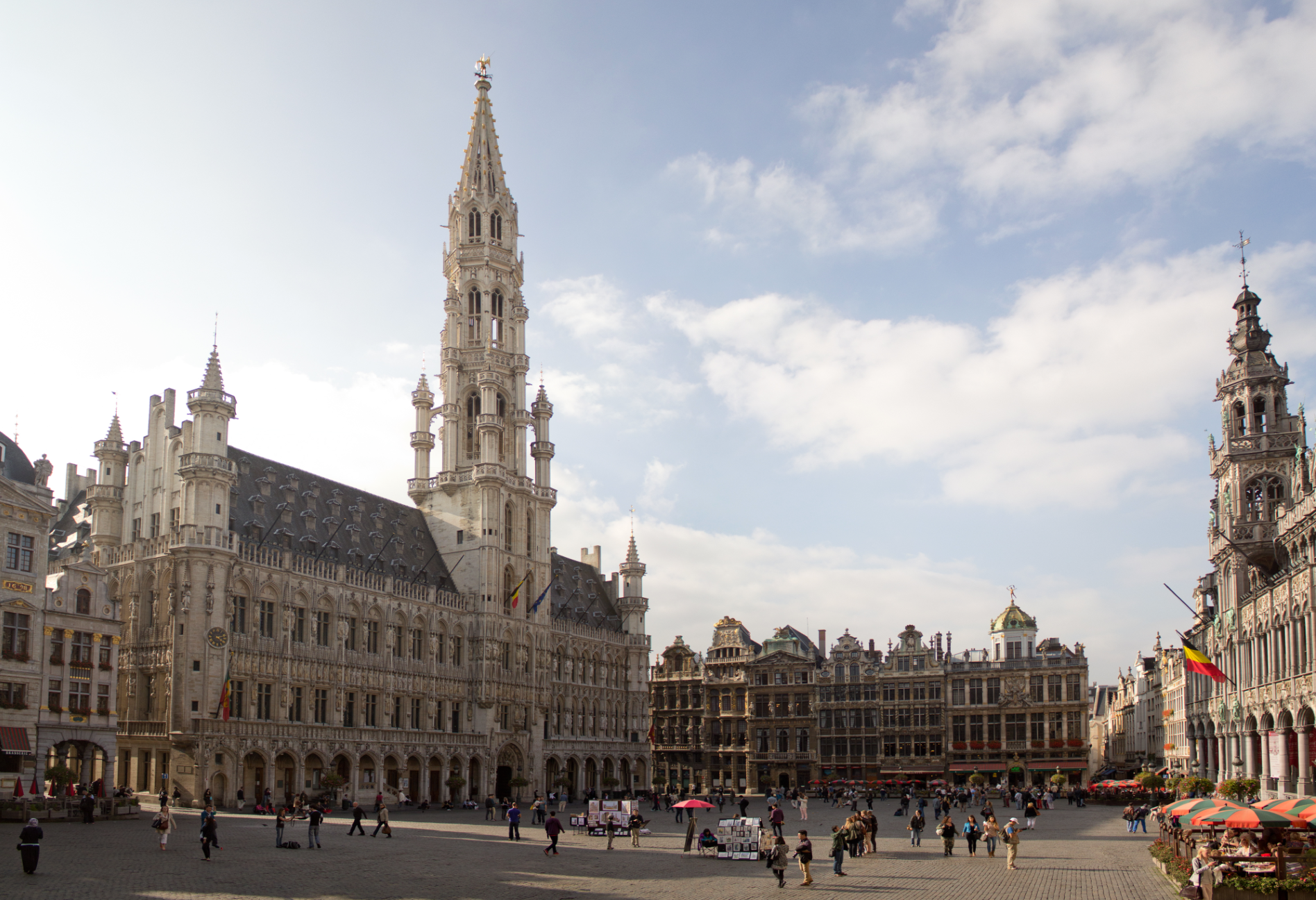# and for the capital BrusselsOur Mission:

to the forefront
of international research
and to stabilize this position.

2. To strengthen cooperation
with international research centers:
• Harish-Chandra Research Institute,
• Washington and Lee University,
Lexington, Virginia, USA
• Université Mohammed Premier,
Oujda, Morocco
• Australian National University,
Canberra, Capital Territory
• Aichi University of Education,
Nagoya, Japan
• University of Calgary,

International Conferences:

January 21 - 25, 2019:
Asia-Australia Algebra Conference 2019
Western Sydney University, Parramatta City campus
Sydney, New South Wales, Australia

April 25 - 27, 2019:
Conference on Algebra, Number Theory and Their Applications
Université Mohammed Premier, Faculté des Sciences
Oujda, Region Oriental, Morocco
Daniel C. Mayer's 3 Lectures:
1. Proving the Conjecture of Scholz
2. Differential principal factors
3. Pure Metacyclic Fields

July 1 - 5, 2019:
31st Journées Arithmétiques
Istanbul University, Faculty of Science
JA 2019, Istanbul, TurkeyProgressive Innovations and Outstanding Scientific Achievements:

Unexpected Discoveries on 26 April 2018

1. Although it seems to be an arithmetic invariant,
the degree [Fp(K):K] of the maximal unramified pro-p extension Fp(K)
over an algebraic number field K with assigned Artin transfer pattern AP(K)=(τ(K),κ(K))
cannot be determined by number theoretic methods in general.
The group theoretic strategy of pattern recognition must be employed
on a bounded root region of a suitable descendant tree of finite non-abelian p-groups.

During the CSASC Conference in 2011 at the Danube University Krems,
I presented the Principalization Algorithm via Class Group Structure
for quadratic number fields K=Q(d1/2) with non elementary 3-class group Cl3(K)∼(21),
which is based on the fact that, for certain Artin patterns,
the components τ(K) and κ(K) determine each other uniquely
(τ(K) denotes the abelian quotient invariants (AQI) of first order
and κ(K) denotes the punctured transfer kernel type (pTKT) of K).

This correspondence is expressed by the following Theorem:
τ(K)∼((31)2,(212)2) if and only if κ(K)∼(124;1), called type D.11,
τ(K)∼((31)3,212) if and only if κ(K)∼(123;4), called type E.12,
τ(K)∼((31)3,14) if and only if κ(K)∼(111;4), called type B.7.

Theoretical results were underpinned by explicit computation of all Artin patterns
for discriminants in the range -106 < d < 107.

2. In 2015, Michael R. Bush extended my range of discriminants considerably to -108 < d < 109
classifying by the first component τ(K) and abstaining from the fine structure splitting by means of κ(K).
A comparison of the above mentioned three pTKTs with respect to the distribution of their absolute frequencies
in four partial ranges of discriminants reveals a very dense population for imaginary quadratic fields:

 pTKT -105 < d < 0 -106 < d < 0 -107 < d < 0 -108 < d < 0 -108 < d < 0 D.11 14 406 4974 55310 45.2% E.12 13 75 844 9335 7.6% B.7 3 64 799 9000 7.4% total 48 875 10811 122444 100%

3. However, for real quadratic fields, the population turns out to be rather sparse:

 pTKT 0 < d < 106 0 < d < 107 0 < d < 108 0 < d < 109 0 < d < 109 D.11 1 16 221 2844 4.8% E.12 0 3 34 517 0.9% B.7 0 2 35 489 0.8% total 12 271 4679 59081 100%

4. For the punctured transfer kernel type D.11,
the two possible metabelian 3-groups Gal(F32(K)/K) ∼ <729,i> with i∈{14,15}
are Schur σ-groups, whence F3(K)=F32(K) is of degree 729 over K.

Theorem: The following three conditions are equivalent:
(1) τ(K)∼((31)2,(212)2),
(2) κ(K)∼(124;1), called type D.11,
(3) Gal(F3(K)/K) ∼ <729,i> with i∈{14,15}.

5. Now I come to the unexpected discoveries:

Theorem: Let K=Q(d1/2) be a quadratic field with
3-class group Cl3(K)∼(21) and abelian quotient invariants of first order τ(K)∼((31)3,212)
(which is equivalent to a punctured transfer kernel type κ(K)∼(123;4), called type E.12).
Then abelian quotient invariants of second order τ(2)(K) can be used for the following criteria:

(1) If Gal(F3(K)/K) ∼ <2187,178>-#2;2 of order 19683, then
Gal(F32(K)/K) ∼ <6561,1734> and
τ(2)(K)∼ (21;[(31);221,(41)3]3,[212;221,(312)6,(32)6]).

(2) If Gal(F3(K)/K) ∼ <2187,188>-#2;2 of order 19683, then
Gal(F32(K)/K) ∼ <6561,1772> and
τ(2)(K)∼ (21;[(31);221,(41)3]3,[212;221,(32)12]).

(3) τ(2)(K)∼ (21;[(31);221,(41)3],[(31);221,(31)3]2,[212;221,(212)6,(22)6])
if and only if K is real with d > 0 and
Gal(F3(K)/K) ∼ <6561,1735>, Gal(F32(K)/K) ∼ <2187,178>.

(4) τ(2)(K)∼ (21;[(31);221,(41)3],[(31);221,(31)3]2,[212;221,(22)12])
if and only if K is real with d > 0 and
Gal(F3(K)/K) ∼ <6561,1773>, Gal(F32(K)/K) ∼ <2187,188>.

Remark: The criteria in item (3) and (4) of the theorem are necessary and sufficient.
However, the inverse implications in item (1) and (2) of the theorem cannot be proved rigorously,
since counterexamples can occur (observe that, for i∈{178,188}, the Schur σ-groups <2187,i>-#2;2 and
<2187,i>-#2;1-#1;2-#2;2 share common AQI of second order τ(2)(K)
but the latter has a different metabelianization <6561,j>-#1;2 with j∈{1733,1771}).
Nevertheless the inverse implications are true with high probability, in a statistical sense.

6. Examples: The abelian quotient invariants of second order τ(2)(K)∼
(1) (21;[(31);221,(41)3]3,[212;221,(312)6,(32)6]) occur for d∈{-19427,-42591,-49576},
where Gal(F3(K)/K) must be a Schur σ-descendant of <2187,178>,
(2) (21;[(31);221,(41)3]3,[212;221,(32)12]) occur for d∈{-5703,-19919,-27635,57336,-61771},
where Gal(F3(K)/K) must be a Schur σ-descendant of <2187,188>,
(3) (21;[(31);221,(41)3],[(31);221,(31)3]2,[212;221,(212)6,(22)6]) do not occur for 0 < d < 107,
(4) (21;[(31);221,(41)3],[(31);221,(31)3]2,[212;221,(22)12]) occur for d∈{1893032,9682121,9698952},
where Gal(F3(K)/K) ∼ <6561,1773> is determined uniquely.
(These three are the only real quadratic fields of type E.12 with discriminant 0 < d < 107.)

Preprints of peer reviewed publications:

Isoclinic propagation of algebraic invariants

Co-periodicity isomorphisms between forests of finite p-groups

Successive approximation of p-class towers

Deep transfers of p-class tower groups

Modeling rooted in-trees by finite p-groups

Recent progress in determining p-class field towersPrincipal Investigator and